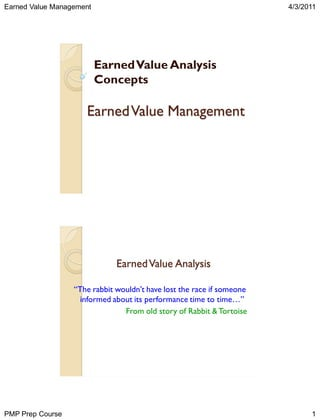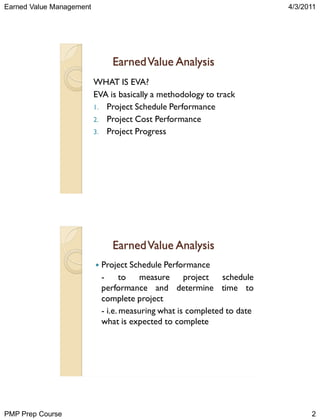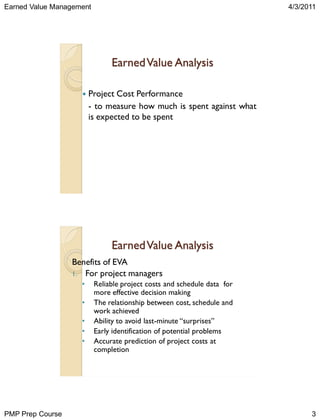Se está descargando tu SlideShare. ×

# Project managment 6 (a)

Anuncio
Anuncio
Anuncio
Anuncio
Anuncio
Anuncio
Anuncio
Anuncio
Anuncio
Anuncio
AnuncioAnuncio
Anuncio
Cargando en…3
×

1 de 14 Anuncio

# Project managment 6 (a)

Anuncio
Anuncio

### Project managment 6 (a)

1. 1. Earned Value Management 4/3/2011 Earned Value Analysis Concepts Earned Value Management Earned Value Analysis “The rabbit wouldn’t have lost the race if someone informed about its performance time to time…” From old story of Rabbit & Tortoise PMP Prep Course 1
2. 2. Earned Value Management 4/3/2011 Earned Value Analysis WHAT IS EVA? EVA is basically a methodology to track 1. Project Schedule Performance 2. Project Cost Performance 3. Project Progress Earned Value Analysis  PMP Prep Course Project Schedule Performance to measure project schedule performance and determine time to complete project - i.e. measuring what is completed to date what is expected to complete 2
3. 3. Earned Value Management 4/3/2011 Earned Value Analysis  Project Cost Performance - to measure how much is spent against what is expected to be spent Earned Value Analysis Benefits of EVA 1. For project managers • • • • • PMP Prep Course Reliable project costs and schedule data for more effective decision making The relationship between cost, schedule and work achieved Ability to avoid last-minute “surprises” Early identification of potential problems Accurate prediction of project costs at completion 3
4. 4. Earned Value Management 4/3/2011 Earned Value Analysis Benefits of EVA 2. Provide ability to answer ◦ ◦ ◦ ◦ ◦ What did we get for money spent How much will the project cost to complete When will the project be complete Which activities are contributing to the cost overrun Which resources contributing to the schedule slippage Earned Value Analysis Planned Value (PV) Planned Value (PV). PV tells you what you plan to do. Planned Value = Physical Work + Approved Budget PV, also known as Budgeted Cost of Work Scheduled (BCWS) PV is categorized as: Cumulative PV is the sum of the approved budget for activities scheduled to be performed to date. Current PV is the approved budget for activities scheduled to be performed during a given period. PMP Prep Course 4
5. 5. Earned Value Management 4/3/2011 Earned Value Analysis PV example We are working on a Client/Server project, and part of the scope is for Software Design. The time frame is 5 months and the budget for this scope is \$15,000, resulting in a budget of \$3,000 per month. Client/Server project – Software Design Dollars PV JAN 3000 FEB 3000 MAR 3000 APR 3000 What is current and cumulative PV? MAY 3000 As on today Earned Value Analysis The Cumulative PV is the total for the elapsed months: January – March. The cumulative PV is \$9,000. The Current PV is the budget for the current month, March, and equals \$3,000. Client/Server project – Software Design Dollars PV JAN 3000 FEB 3000 MAR 3000 APR 3000 MAY 3000 As on today PMP Prep Course 5
6. 6. Earned Value Management 4/3/2011 Earned Value Analysis Budget at Completion (BAC) BAC is the sum of all budgets allocated to a project scope. ◦ BAC can be obtained by work packages ◦ The Project BAC must always equal the Project Total PV. ◦ If they are not equal, your earned value calculations and analysis will be inaccurate. Earned Value Analysis What is the BAC for this project if Software Design is the complete scope of the project? Client/Server project – Software Design Dollars PV JAN 3000 FEB 3000 MAR 3000 APR 3000 MAY 3000 As on today •Yes, BAC = \$15,000. And, in keeping with the previous points about BAC •The project BAC equals the Project Total PV. •The Earned Value calculations are correct. PMP Prep Course 6
7. 7. Earned Value Management 4/3/2011 Earned Value Analysis Actual Cost (AC) Actual Cost (AC), also called actual expenditures, is the cost incurred for executing work on a project.. AC is also called Actual Cost of Work Performed (ACWP). Cumulative AC is the sum of the actual cost for activities performed to date. Current AC is the actual costs of activities performed during a given period. Earned Value Analysis Cumulative AC is the sum of the actual cost for activities performed to date, and Current AC is the actual costs of activities performed during a given period. Client/Server project – Software Design PV AC Dollars JAN 3000 1100 FEB 3000 1200 MAR 3000 1500 APR 3000 MAY 3000 As on today •The Cumulative AC is the total for the elapsed months: January – March. The Cumulative AC is \$3,800. •The Current AC is the actual cost for the current month, March, and equals \$1,500. PMP Prep Course 7
8. 8. Earned Value Management 4/3/2011 Earned Value Analysis Earned Value (EV) EV is the quantification of the “worth” of the work done to date. EV tells us, in physical terms, what the project has accomplished. Cumulative EV is the sum of the budget for the activities accomplished to date. Current EV is the sum of the budget for the activities accomplished in a given period. Earned Value Analysis Earned Value example Client/Server project – Software Design Dollars PV AC EV JAN 3000 1100 900 FEB 3000 1200 1000 MAR 3000 1500 1200 APR 3000 MAY 3000 As on today • The Current EV is the sum of the budget for the activities accomplished in the current month, March, and equals \$1,200. • The Cumulative EV is the sum of the budget for the activities accomplished to date: January – March. The cumulative EV is therefore \$3,100. PMP Prep Course 8
9. 9. Earned Value Management 4/3/2011 Earned Value Analysis Client/Server project – Software Design Dollars PV AC EV JAN 3000 1100 900 Cum PV = \$9,000 Cum AC = \$3,800 Cum EV = \$3,100 FEB 3000 1200 1000 MAR 3000 1500 1200 APR 3000 MAY 3000 As on today Current PV = \$3,000 Current AC = \$1,500 Current EV = \$1,200 BAC = \$15,000 Earned Value Analysis Actual Cost (AC) Budget At Completion (BAC) Planned Value (PV) Earned Value (EV) As On Date PMP Prep Course 9
10. 10. Earned Value Management 4/3/2011 Earned Value Analysis   Schedule Variance (SV) = (BCWP – BCWS) = (EV – PV) ◦ A comparison of amount of work performed during a given period of time to what was scheduled to be performed. ◦ A negative variance means the project is behind schedule   Cost Variance (CV) = (BCWP – ACWP) = (EV – AC) ◦ A comparison of the budgeted cost of work performed with actual cost. ◦ A negative variance means the project is over budget. Earned Value Analysis  Schedule Performance Index (SPI) SPI=BCWP/BCWS = EV/PV  SPI<1 means project is behind schedule   Cost Performance Index (CPI) CPI= BCWP/ACWP = EV/AC  CPI<1 means project is over budget   Cost PMP Prep Course Schedule Index (CSI) 10
11. 11. Earned Value Management 4/3/2011 Earned Value Analysis CSI=CPI x SPI  CSI <1, the project is over budget and behind schedule  Estimate At Completion (EAC)  EAC = BAC/CPI  Estimate to Complete (ETC)  ETC = EAC – BAC  Earned Value Analysis To-Complete Performance Index (TCPI)  Is calculated projection of cost performance, must be achieved on the remaining work  That is ratio between remaining work and funds remaining  TCPI = (BAC-EV)/(BAC-AC)  PMP Prep Course 11
12. 12. Earned Value Management 4/3/2011 Earned Value Analysis A \$10,000 software project is scheduled for 4 weeks. At the end of the third week, the project is 50% complete and the actual costs to date is \$9,000 Planned Value (PV) = \$7,500 Earned Value (EV) = \$5,000 Actual Cost (AC) = \$9,000 Earned Value Analysis Schedule Variance = EV – PV = \$5,000 – \$7,500 = - \$2,500  Schedule Performance Index (SPI) = EV/PV = \$5,000 / \$7,500 = 0.66  Cost Variance = EV – AC = \$5,000 - \$9,000 = - \$4,000  Cost Performance Index (CPI) = EV/AC = \$5,000 / \$9,000 = 0.55 The metrics indicate the project is behind schedule and over budget.  On-target projects have an SPI and CPI of 1 or greater  PMP Prep Course 12
13. 13. Earned Value Management 4/3/2011 Earned Value Analysis If the project continues at the current performance, what is the true cost of the project?  Estimate At Complete  = Budget At Complete (BAC) / CPI = \$10,000 / 0.55 = \$18,181 At the end of the project, the total project costs will be \$18,181 Earned Value Analysis Formulas to recap: SCHEDULE COST SV = EV – PV SV = BCWP – BCWS CV = EV – AC CV = BCWP – ACWP SPI = EV / PV SPE = BCWP / BCWS CPI = EV / AC CPI = BCWP / ACWP CSI = CPI * SPI TCPI = (BAC-EV)/(BAC-AC) PMP Prep Course EAC = BAC / CPI ETC = EAC - BAC 13
14. 14. Earned Value Management 4/3/2011 Questions ? PMP Prep Course 14